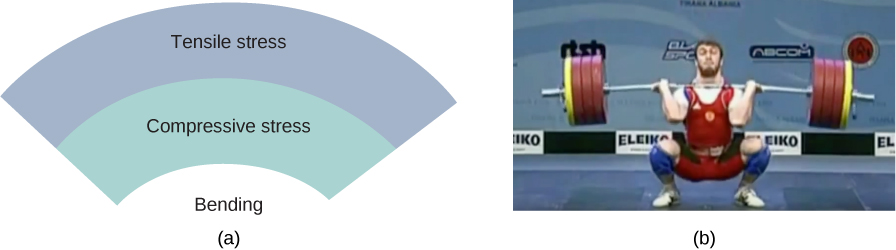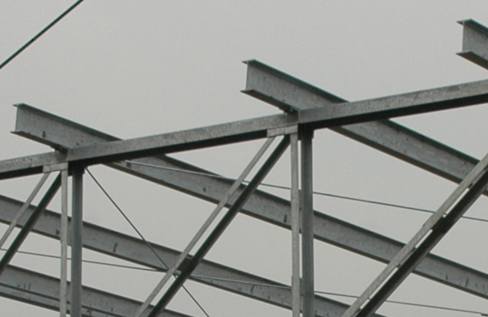# 12.3 Stress, strain, and elastic modulus  (Page 4/26)

 Page 4 / 26

Check Your Understanding Find the compressive stress and strain at the base of Nelson’s column.

$206.8\phantom{\rule{0.2em}{0ex}}\text{kPa};\phantom{\rule{0.2em}{0ex}}4.6\phantom{\rule{0.2em}{0ex}}×\phantom{\rule{0.2em}{0ex}}{10}^{-5}$

## Stretching a rod

A 2.0-m-long steel rod has a cross-sectional area of $0.30\phantom{\rule{0.2em}{0ex}}{\text{cm}}^{2}.$ The rod is a part of a vertical support that holds a heavy 550-kg platform that hangs attached to the rod’s lower end. Ignoring the weight of the rod, what is the tensile stress in the rod and the elongation of the rod under the stress?

## Strategy

First we compute the tensile stress in the rod under the weight of the platform in accordance with [link] . Then we invert [link] to find the rod’s elongation, using ${L}_{0}=2.0\phantom{\rule{0.2em}{0ex}}\text{m}.$ From [link] , Young’s modulus for steel is $Y=\phantom{\rule{0.2em}{0ex}}2.0\phantom{\rule{0.2em}{0ex}}×\phantom{\rule{0.2em}{0ex}}{10}^{11}\text{Pa}.$

## Solution

Substituting numerical values into the equations gives us

$\begin{array}{ccc}\hfill \frac{{F}_{\perp }}{A}& =\hfill & \frac{\left(550\phantom{\rule{0.2em}{0ex}}\text{kg}\right)\left(9.8\phantom{\rule{0.2em}{0ex}}{\text{m/s}}^{2}\right)}{3.0\phantom{\rule{0.2em}{0ex}}×\phantom{\rule{0.2em}{0ex}}{10}^{-5}\phantom{\rule{0.2em}{0ex}}{\text{m}}^{2}}=1.8\phantom{\rule{0.2em}{0ex}}×\phantom{\rule{0.2em}{0ex}}{10}^{8}\text{Pa}\hfill \\ \hfill \text{Δ}L& =\hfill & \frac{{F}_{\perp }}{A}\phantom{\rule{0.2em}{0ex}}\frac{{L}_{0}}{Y}=\left(1.8\phantom{\rule{0.2em}{0ex}}×\phantom{\rule{0.2em}{0ex}}{10}^{8}\text{Pa}\right)\phantom{\rule{0.2em}{0ex}}\frac{2.0\phantom{\rule{0.2em}{0ex}}\text{m}}{2.0\phantom{\rule{0.2em}{0ex}}×\phantom{\rule{0.2em}{0ex}}{10}^{11}\text{Pa}}=1.8\phantom{\rule{0.2em}{0ex}}×\phantom{\rule{0.2em}{0ex}}{10}^{-3}\text{m}=\phantom{\rule{0.2em}{0ex}}1.8\phantom{\rule{0.2em}{0ex}}\text{mm.}\hfill \end{array}$

## Significance

Similarly as in the example with the column, the tensile stress in this example is not uniform along the length of the rod. Unlike in the previous example, however, if the weight of the rod is taken into consideration, the stress in the rod is largest at the top and smallest at the bottom of the rod where the equipment is attached.

Check Your Understanding A 2.0-m-long wire stretches 1.0 mm when subjected to a load. What is the tensile strain in the wire?

$5.0\phantom{\rule{0.2em}{0ex}}×\phantom{\rule{0.2em}{0ex}}{10}^{-4}$

Objects can often experience both compressive stress and tensile stress simultaneously [link] . One example is a long shelf loaded with heavy books that sags between the end supports under the weight of the books. The top surface of the shelf is in compressive stress and the bottom surface of the shelf is in tensile stress. Similarly, long and heavy beams sag under their own weight. In modern building construction, such bending strains can be almost eliminated with the use of I-beams [link] .(a) An object bending downward experiences tensile stress (stretching) in the upper section and compressive stress (compressing) in the lower section. (b) Elite weightlifters often bend iron bars temporarily during lifting, as in the 2012 Olympics competition. (credit b: modification of work by Oleksandr Kocherzhenko)Steel I-beams are used in construction to reduce bending strains. (credit: modification of work by “US Army Corps of Engineers Europe District”/Flickr)

A heavy box rests on a table supported by three columns. View this demonstration to move the box to see how the compression (or tension) in the columns is affected when the box changes its position.

## Bulk stress, strain, and modulus

When you dive into water, you feel a force pressing on every part of your body from all directions. What you are experiencing then is bulk stress, or in other words, pressure    . Bulk stress always tends to decrease the volume enclosed by the surface of a submerged object. The forces of this “squeezing” are always perpendicular to the submerged surface [link] . The effect of these forces is to decrease the volume of the submerged object by an amount $\text{Δ}V$ compared with the volume ${V}_{0}$ of the object in the absence of bulk stress. This kind of deformation is called bulk strain and is described by a change in volume relative to the original volume:

what is vector
How to calculate for overall displacement
Well, take Final Position-Starting position
Grant
what is velocity
speed per unit time is called velocity. it is a vector quantity
Mukulika
velocity is distances overall time taking,it is a vector quantity, the units is metre per second.
Samuel
frequency is rate at which something happens or is repeated. it is a vector quantity
mawuo
what is the difference between resultant force and net force
net force is when you add forces numerically I.e. the total sum of all positive and negative or balanced and unbalanced forces. resultant force is a single vector which is the combination or addition of all x and y axes vector component forces in a system.
emmanuel
thanks
Ogali
resultant force is applied to hold or put together an object moving at the wrong direction. in other words it repairs.
Andrew
Damping is provided by tuning the turbulence levels in the moving water using baffles.How it happens? Give me a labelled diagram of it.
A 10kg ball travelling at 4meter per second collides elastically in a head-on collision with a 2kg ball.What are (a)the velocities and (b)the total momentum of the balls after collision?
a)v1 8/3s&v2 20/3s. b)in elastic collision total momentum is conserved.
Bala
multiply both weight which is 10*2 divided by the time give 4. and our answer will be 5.
Andrew
The displacement of the air molecules in sound wave is modeled with the wave function s(x,t)=5.00nmcos(91.54m−1x−3.14×104s−1t)s(x,t)=5.00nmcos(91.54m−1x−3.14×104s−1t) . (a) What is the wave speed of the sound wave? (b) What is the maximum speed of the air molecules as they oscillate in simple harmon
the question is wrong. if you need assistance with displacement I can help out.
Andrew
practical 1st year physics
huh
Luminous
allot of practicals, be specific with your topic and we can discuss.
Andrew
Whats the formular for newton law of motion
f=ma
F=m×a Where F=force M=mass of a body of an object a=acceleration due to gravity
Abubakar
what is speed
distance travelled per unit of time is speed.
distance travelled in a particular direction it is.
Andrew
Speed is define as the distance move per unit time. Mathematically is given as Speed = distance/time Speed = s/t
Abubakar
speed is a vector quantity. It is defined distance per unit time.It's unit in c.g.s cm/s and in S.I. m/s.It’s dimension is LT^-1
Mukulika
formula for velocity
v=ms^-1 velocity=distance time
Cleophas
(p-a/v)(v-b)=nrt what is the dimension of a
Amraketa
velocity= displacement time
Gold
Velocity = speed/time
Abubakar
what are evasive medical diagnosis?
If the block is displaced to a position y , the net force becomes Fnet=k(y−y0)−mg=0Fnet=k(y−y0)−mg=0 . But we found that at the equilibrium position, mg=kΔy=ky0−ky1mg=kΔy=ky0−ky1 . Substituting for the weight in the equation yields. Show me an equation of graph.
Shaina
where are you come from
Lida
samastipur Bihar
carrier
simple harmonic motion defination
how to easily memorize motion equation
Maharam
how speed destrog is uranium
where can we find practice problems?
I'm not well
YAZID
SayedByByByBy David MartinBy